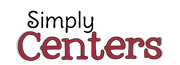## Thursday, February 12, 2015

### Milk Lid MathStart saving those milk jug lids because there are countless math activities you can do in your classroom using this free manipulative
Here are just four of those ideas.

1) Sort the lids by various attributes such as:
• Color
• Snap-on or Twist-on
• Label or No Label
• Kind of edge (smooth or rough)

2) Let the students grab one handful of lids.
• Ask the students to count the lids.
• See if the students can write that number.

3) Make a pattern using two different colors of lids.

• Identify the pattern using letters of the alphabet or numbers. The pattern above would be an A, A, B pattern or a 1, 1, 2 pattern.
• Now ask the students to use more than two colors to make a pattern
• Once more, have the students identify the pattern using alphabet letters or numbers.

4) Decide on a money value for each color of lid. (Example: Red lids are worth a nickel, blue lids are worth a dime, and white lids are worth a penny.) Put all of the lids into a bag and have the students draw out four lids. Have the students add up the total value of these four lids.Milk Lid Math
• Use play money (coins) to have the students show the value of the lids.
• Have the students practice writing money as either a part of a dollar or as cents.
• Another idea is to have the students find all the combinations of lids that would equal a nickel or a dime or a quarter.

On the original download you will receive 15 ideas with numerous subtopics listed under each one. These may be used with a whole group, small groups, or as center activities.